# Magneto-fluid dynamics

(diff) ← Older revision | Latest revision (diff) | Newer revision → (diff)
The study of complicated phenomena of motion of a liquid or a gaseous conducting medium under the influence of an external magnetic field. The hydrodynamic flows of the medium induce electric fields and generate electric currents, which in their turn experience the influence of the magnetic field as well as change it. This interaction of electromagnetic and hydrodynamic phenomena is described by a combined system of field equations and equations of fluid dynamics. For an ideal liquid (the effects related with viscosity and heat conduction are not taken into account, the conductivity of the medium is assumed to be infinitely large and the magnetic permeability is set equal to), the complete system of equations of magneto-fluid dynamics has the form (see, e.g., [a1], Sect. 65)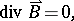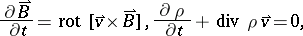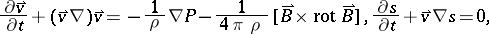where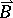is the magnetic field,is the density of the liquid,is the entropy of a liquid mass unit, andis the pressure, which is related to the densityof the liquid and its temperaturethrough a state equation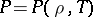.